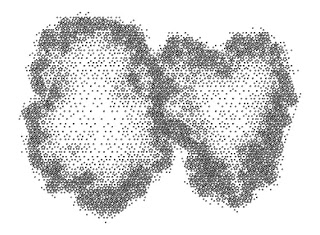## Thursday, June 10, 2010

### Self-Similar Vertices, Part 2

This is the second of two methods that I use to create dot patterns with self-similar tile sets. My interest in tilings is relative to art, not math. These tilings, or tile patches, are one solution to a problem I encountered while generating tilings as structures for a series of vertex pattern diagrams. I needed a way to create greater density changes than I was getting with other tilings. Self-similar tile sets solve the problem.The tile set described in Part 1 is closely related to, and can be derived from tilings described by Robert W. Fathauer. Fathauer found two families of self-similar tilings based on segments of regular polygons (see Reference below). One family includes an 18-18-144 triangle that is a segment of a regular decagon. The triangle is the s=10 prototile described by Fathauer. The tile set that I use includes a pentagon as well as the triangle. The pentagon is the shape that remains after removing five s=10 tiles from a regular decagon. The tile set I describe here in Part 2 is also self-similar, but doesn’t include Fathauer’s prototiles.

The method I describe here is based on an infinitely self-similar tile set consisting of a partially concave hexagon and two isosceles triangle prototiles. The hexagon is congruent to a regular pentagon except one side of the pentagon has been made concave with two smaller sides forming interior angles of 72, 252, and 72 degrees in addition to three 108 degree interior angles. The triangles are 72-72-36 and 36-36-108 isosceles triangles.

The initial hexagon and triangle prototiles are sized so the two long sides of the 72-72-36 triangle are equal to the long sides of the hexagon. The single long side of the 36-36-108 triangle is also equal to the long sides of the hexagon. The 36-36-108 triangle fits exactly in the concave area of the hexagon, and together the 36-36-108 triangle and the hexagon form a regular pentagon. The tile set in Part 1 was infinitely scalable pentagon-triangle pairs by setting the next pentagon side equal to the short side of the previous triangle. The tile set describe here is infinitely scalable by setting the next hexagon long sides equal to a short side of the previous pentagon.Tilings should be edge-to-edge, with no overlaps. Gaps are always fillable. Singularities are not necessary. Tilings may not fill the plane, but could be infinitely scalable at the boundaries, as in a fractal. These are actually tile patches, not tessellations. A radially symmetrical tiling of just hexagons looks like the following figure.

The tilings are a structure for the finished diagram of vertices and what I call extrinsic vertices. This demonstrates the advantage of using a tiling with properties of self-similarity. The changing density of tiles translates to a greater variation in vertex density.

It's possible to create numerous symmetrical tilings with these tiles, but I often choose to create asymmetrical diagrams. The processes, lattices, and patterns I use are not math. I'm influenced by structures in math, science, architecture, and design, but unconstrained by the rigorousness of math. These diagrams have no practical use or purpose other than art.

Reference

Fathauer, Robert W. (2000). "Self-similar Tilings Based on Prototiles Constructed from Segments of Regular Polygons," presented at the Bridges Conference (July 28-30, 2000, Southwestern College, Winfield, Kansas).
http://www.mathartfun.com/shopsite_sc/store/html/Compendium/Bridges2000.pdf
Also see:
http://www.mathartfun.com/shopsite_sc/store/html/Compendium/encyclopedia.html